## 概述

Tacotron2是由Google Brain 2017年提出来的一个语音合成框架Tacotron2:一个完整神经网络语音合成方法。模型主要由三部分组成：
• 声谱预测网络：一个引入注意力机制（attention）的基于循环的Seq2seq的特征预测网络，用于从输入的字符序列预测梅尔频谱的帧序列。
• 声码器（vocoder）：一个WaveNet的修订版，用预测的梅尔频谱帧序列来生成时域波形样本。
• 中间连接层：使用低层次的声学表征-梅尔频率声谱图来衔接系统的两个部分。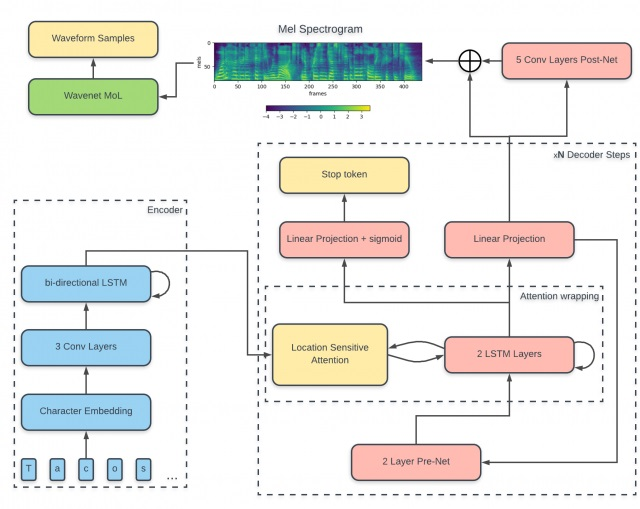## 预处理

### 文字处理

1. 先清除文字里陌生字符， 可以使用正则表达式匹配

_curly_re = re.compile(r'(.*?)\{(.+?)\}(.*)')

2. 对每个字母进行编码, 得到一个词向量， 然后传给模型

 _pad = '_'
_punctuation = '!\'(),.:;? '
_special = '-'
_letters = 'ABCDEFGHIJKLMNOPQRSTUVWXYZabcdefghijklmnopqrstuvwxyz'

# Prepend "@" to ARPAbet symbols to ensure uniqueness:
_arpabet = ['@' + s for s in cmudict.valid_symbols]

# Export all symbols:
symbols = [_pad] + list(_special) + list(_punctuation) + list(_letters) + _arpabet

def sequence_to_text(sequence):
result = ''
for symbol_id in sequence:
if symbol_id in _id_to_symbol:
s = _id_to_symbol[symbol_id]
if len(s) > 1 and s == '@':
s =  s[1:]
result += s
return result.replace('}{', ' ')


### 声音处理

from scipy.io.wavfile import read
sampling_rate, data = read(full_path)


from scipy.signal import get_window

# get window and zero center pad it to filter_length
fft_window = get_window(window, win_length, fftbins=True)
fft_window = pad_center(fft_window, filter_length)
fft_window = torch.from_numpy(fft_window).float()
forward_basis *= fft_window
inverse_basis *= fft_window
# 加窗
forward_transform = F.conv1d(
input_data,
Variable(self.forward_basis, requires_grad=False),
stride=self.hop_length, padding=0)
# 短时傅里叶变换
cutoff = int((self.filter_length / 2) + 1)
real_part = forward_transform[:, :cutoff, :]  # 实部
imag_part = forward_transform[:, cutoff:, :]  # 虚部
magnitude = torch.sqrt(real_part ** 2 + imag_part ** 2)
mel_output = torch.matmul(self.mel_basis, magnitudes)


## 傅里叶变换

• A表示频率为0hz的信号，即直流分量

• A[1:N/2]包含正频率项，A[N/2:]包含负频率项。正频率项就是转化后的频域信号，通常我们只需要正频率项，即前面的n/2项，负频率项是计算的中间结果（正频率项的镜像值）

• A[i] = real + j * imag，是一个复数，相位就是复数的辐角，相位 = arg(real/imag)

• 振幅就是复数的模，振幅 = $\sqrt{real^2+imag^2}$。但是fft的返回值的模是放大值，直流分量的振幅放大了N倍，弦波分量的振幅放大了N/2倍

import numpy as np
from scipy.fftpack import fft, ifft
import matplotlib.pyplot as plt

x = np.linspace(0, 1, 800)

# 设置需要采样的信号，频率分量有80，190和300
y = 7 * np.sin(2 * np.pi * 80 * x) + \
2.8 * np.sin(2 * np.pi * 190 * x) + \
5.1 * np.sin(2 * np.pi * 300 * x)

yy = fft(y)  # 快速傅里叶变换
y_real = yy.real  # 获取实数部分
y_imag = yy.imag  # 获取虚数部分

yf = abs(fft(y))  # 取绝对值
yf1 = 2 * abs(fft(y)) / len(x)  # 归一化处理
yf2 = yf1[range(int(len(x) / 2))]  # 由于对称性，只取一半区间

xf = np.arange(len(y))  # 频率
xf1 = xf
xf2 = xf[range(int(len(x) / 2))]  # 取一半区间

plt.figure(figsize=(10, 4))
plt.subplot(231)
plt.plot(x[0:50], y[0:50])

plt.subplot(232)
plt.plot(xf, y_real, 'r')

plt.subplot(233)
plt.plot(xf, y_imag, 'g')

plt.subplot(234)
plt.plot(xf1, yf, 'g')

plt.subplot(235)
plt.plot(xf1, yf1, 'r')

plt.subplot(236)
plt.plot(xf2, yf2, 'b')

plt.show()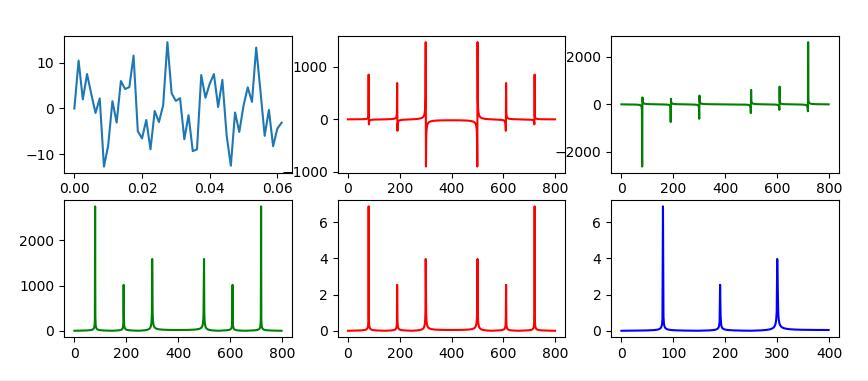(Fig1原始波形，Fig2实数部分, Fig3虚数部分，Fig4绝对值, Fig5归一化，Fig6对称取半)

#### DCT

SciPy.fftpack中，提供了离散余弦变换(DCT)与离散余弦逆变换(IDCT)的实现。我们将上面fft运算改成dct运算，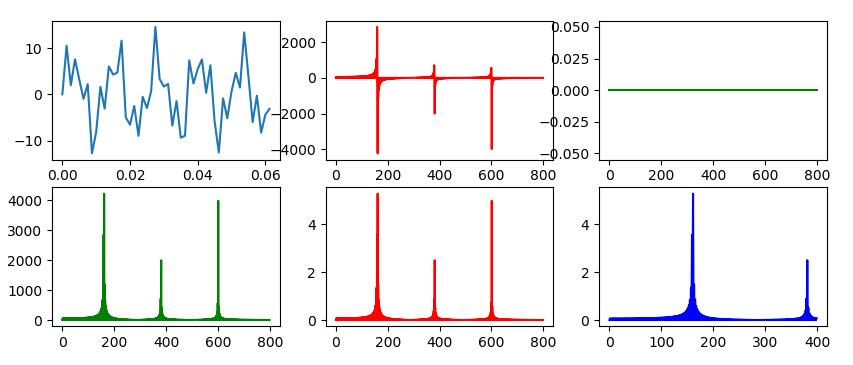## 编码器-解码器(Encoder-Decoder)结构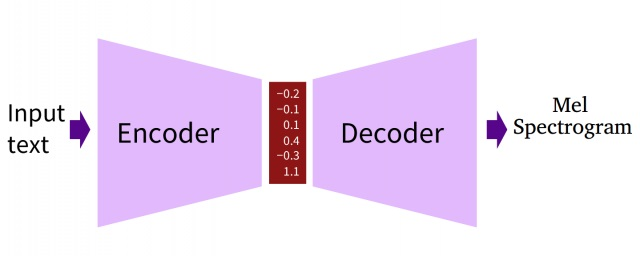## 编码器

### 注意力机制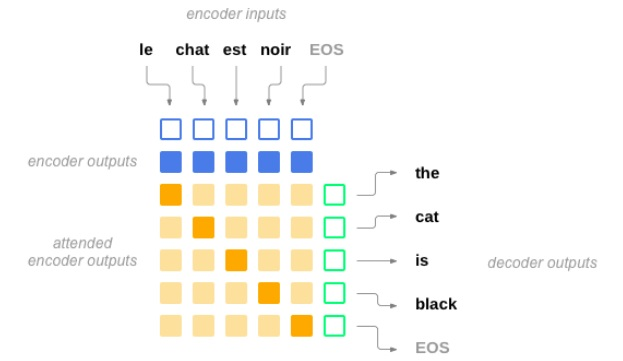##### 一般的计算步骤：

• 点积 $Similarity(Query,Key)=Query·Key$
• cos相似性 $Similarity(Query,Key)=\frac{Query·Key_i}{||Query||*||Key_i||}$
• MLP网络 $Similarity(Query,Key_i)=MLP(Query,Key_i)$
• Key和Value还可以拼接后再内积一个参数向量，甚至权重都不一定要归一化

#### Tacotron2注意力机制，Location Sensitive Attention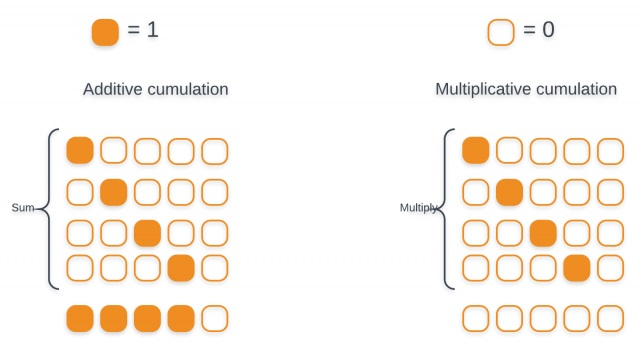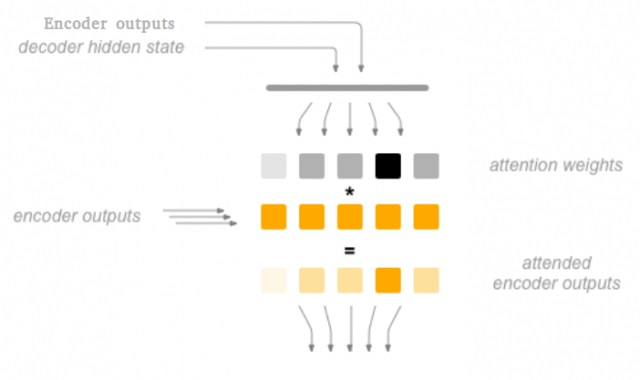## 解码器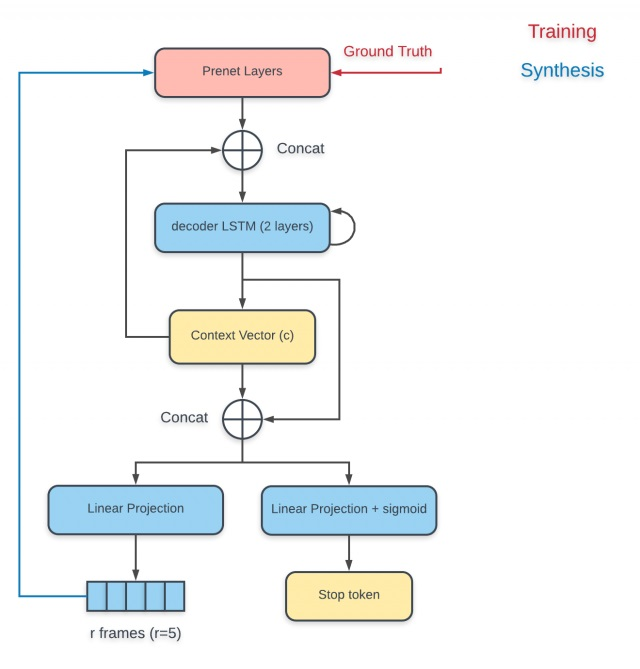PreNet的输出与使用上一个解码步输出计算而得的上下文向量做拼接，然后整个送入RNN解码器中，RNN解码器的输出用来计算新的上下文向量，最后新计算出来的上下文向量与解码器输出做拼接，送入投影层(projection layer)以预测输出。输出有两种形式，一种是声谱帧，一种是的概率，后者是一个简单二分类问题，决定解码过程是否结束。使用缩减因子(reduction factor)即每一个解码步仅允许预测r(缩减因子)Mel谱帧，能够有效加速计算，减小内存占用。